TitleCollege Algebra
Tutorial 9: Multiplying and Dividing Rational ExpressionsLearning Objectives

 After completing this tutorial, you should be able to: Multiply rational expressions. Divide rational expressions.Introduction

 In this tutorial I will be stepping you through how to multiply and divide rational expressions.  A lot of times in math you are having to use past concepts to be able to work all the way through the new problems.  In this section you will have to remember how to factor, simplify rational expressions and multiply polynomials to be able to complete the multiplication or division problems.  If you need a review on multiplying polynomials, feel free to go back to Tutorial 6: Polynomials.  If you need a review on factoring, feel free to go back to Tutorial 7: Factoring Polynomials.   If you need a review on simplifying rational fractions, feel free to go back to Tutorial 8: Simplifying Rational Expressions.   I think that you are ready to move ahead.Tutorial

 Multiplying Rational Expressions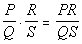Q and S do not equal 0.

 Step 1: Factor both the numerator and the denominator.

 If you need a review on factoring, feel free to go back to Tutorial 7: Factoring Polynomials.

 Step 2: Write as one fraction.

 Write it as a product of the factors of the numerators over the product of the factors of the denominators.  DO NOT multiply anything out at this point.

 Step 3: Simplify the rational expression.

 If you need a review on factoring, feel free to go back to Tutorial 8: Simplifying Rational Expressions.

 Step 4: Multiply any remaining factors in the numerator and/or denominator.

 If you need a review on multiplying polynomials, feel free to go back to Tutorial 6: Polynomials.Example 1:   Multiply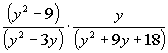.

 Step 1: Factor both the numerator and the denominator AND

 Step 2: Write as one fraction.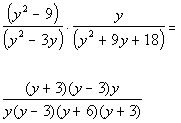*Factor the num. and den.

 In the numerator we factored a difference of squares. In the denominator we factored a GCF and a trinomial.

 Step 3: Simplify the rational expression. AND

 Step 4: Multiply any remaining factors in the numerator and/or denominator.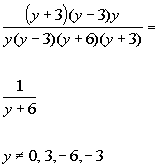*Simplify by div. out the common factors of (y + 3), (y - 3) and y       *Excluded values of the original den.

 Note that even though all of the factors in the numerator were divided out there is still a 1 in there.  It is easy to think there there is "nothing" left and make the numerator disappear.  But when you divide a factor by itself there is actually a 1 there.  Just like 2/2 = 1 or 5/5 = 1.   Also note that the values that would be excluded from the domain are 0, 3, -6, and -3.  Those are the values that makes the original denominator equal to 0.Example 2:   Multiply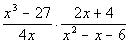.

 Step 1: Factor both the numerator and the denominator AND

 Step 2: Write as one fraction.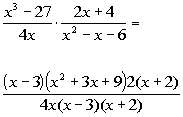*Factor the num. and den.

 In the numerator we factored a difference of cubes and a GCF. In the denominator we factored a  trinomial.

 Step 3: Simplify the rational expression. AND

 Step 4: Multiply any remaining factors in the numerator and/or denominator.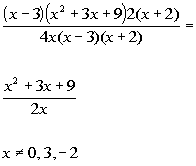*Simplify by div. out the common factors of  (x - 3), 2, and (x + 2)       *Excluded values of the original den.

 Note that the values that would be excluded from the domain are 0, 3, and -2.  Those are the values that makes the original denominator equal to 0.

 Dividing Rational Expressions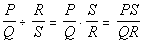where Q, S, and R do not equal 0.

 Step 1: Write as multiplication of the reciprocal.   Step 2: Multiply the rational expressions as shown above.Example 3:   Divide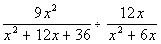.

 Step 1: Write as multiplication of the reciprocal AND  Step 2: Multiply the rational expressions as shown above.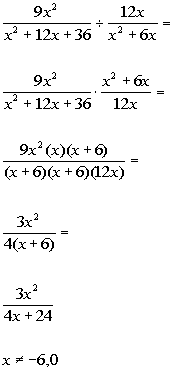*Rewrite as mult. of reciprocal   *Factor the num. and den. *Simplify by div. out the common factors of  3x and (x + 6)         *Multiply the den. out   *Excluded values of the original den. of product

 In the numerator of the product we factored a GCF. In the denominator we factored a  trinomial.

 Note that the values that would be excluded from the domain are -6 and 0.  Those are the values that makes the original denominator of the product equal to 0.Example 4:   Divide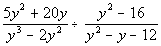.

 Step 1: Write as multiplication of the reciprocal AND  Step 2: Multiply the rational expressions as shown above.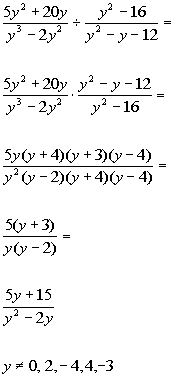*Rewrite as mult. of reciprocal   *Factor the num. and den. *Simplifyby div. out the common factors of  y, (y + 4), and (y - 4)       *Multiply the num. and den. out     *Excluded values of the original den. of quotient & product

 In the numerator of the product we factored a GCF and a trinomial. In the denominator we factored a GCF  and a difference of squares.

 Note that the values that would be excluded from the domain are 0, 2, - 4, 4, and -3.  Those are the values that make the original denominator of the quotient and the product equal to 0.Practice Problems

 These are practice problems to help bring you to the next level.  It will allow you to check and see if you have an understanding of these types of problems. Math works just like anything else, if you want to get good at it, then you need to practice it.  Even the best athletes and musicians had help along the way and lots of practice, practice, practice, to get good at their sport or instrument.  In fact there is no such thing as too much practice. To get the most out of these, you should work the problem out on your own and then check your answer by clicking on the link for the answer/discussion for that  problem.  At the link you will find the answer as well as any steps that went into finding that answer.Practice Problems 1a - 1b: Perform the indicated operation.

 1a.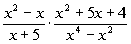(answer/discussion to 1a) 1b.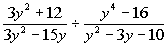(answer/discussion to 1b)Need Extra Help on these Topics?

The following are webpages that can assist you in the topics that were covered on this page:

 http://www.wtamu.edu/academic/anns/mps/math/mathlab/int_algebra/int_alg_tut32_multrat.htm This webpage helps you with multiplying and dividing rational expressions. http://www.purplemath.com/modules/rtnlmult.htm This website helps you with multiplying and dividing rational expressions.

Go to Get Help Outside the Classroom found in Tutorial 1: How to Succeed in a Math Class for some more suggestions.

WTAMU > Virtual Math Lab > College Algebra

Videos at this site were created and produced by Kim Seward and Virginia Williams Trice.
Last revised on Dec. 14, 2009 by Kim Seward.